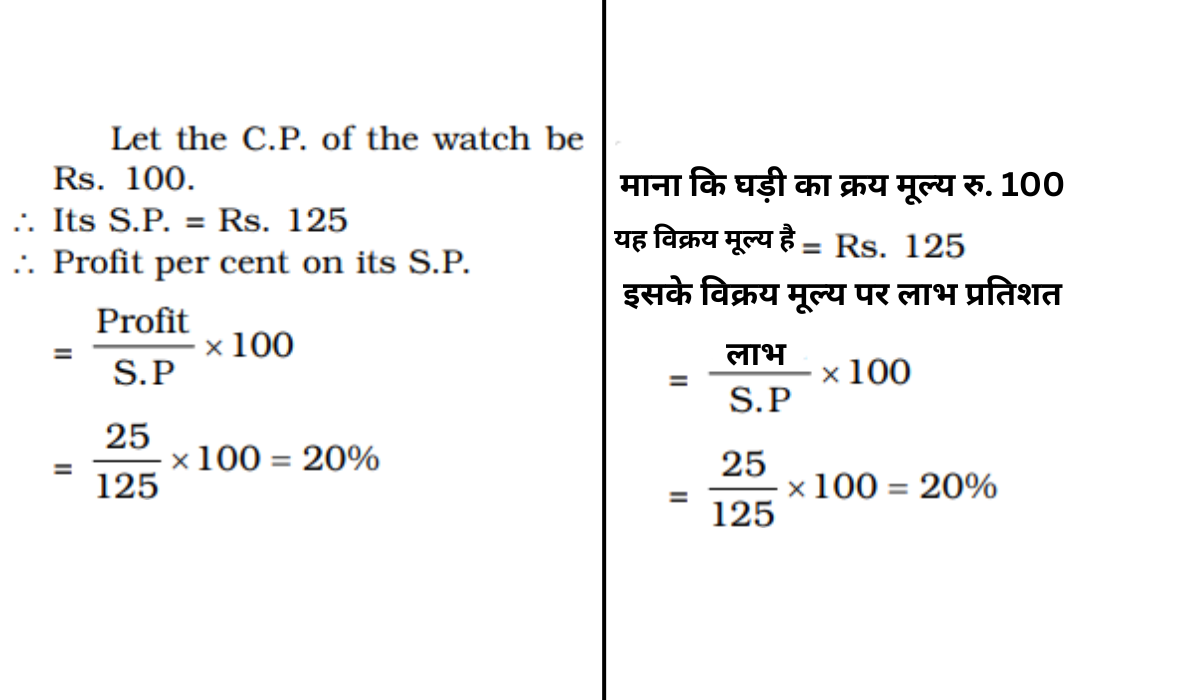• Save

# Profit and Loss Questions and Answers for Competitive Exams

NEWProfit and Loss Questions and Answers for Competitive Exams" is a crucial topic assessing an individual's aptitude in business mathematics. This segment evaluates one's comprehension of financial concepts related to calculating profit, loss, cost price, selling price, and profit percentage. The set of questions typically involves scenarios where candidates are required to determine the cost price, selling price, or profit percentage based on given information. Mastery in this area showcases the ability to analyse business transactions, compute profits or losses accurately, and make informed decisions.

## Profit and Loss Questions

In this article Profit and Loss Questions and Answers for Competitive Exams, we are providing Profit and Loss Questions and Answers under Aptitude and General Math section for upcoming competitive exams like SSC, Bank ,RRB and other government exams.

Also, Read Latest Current Affairs Questions 2023: Current Affairs Today

"Put your knowledge to the test and excel with our General Knowledge Mock Test and Current Affairs Mock Test!"

## Profit and Loss Questions and Answers for Competitive Exams

Q :

The cost price of a radio is Rs. 600. The 5% of the cost price is charged towards transportation. After adding that, If net profit to be made is 15%, then the selling price of the radio must be

(A) Rs. 664.50

(B) Rs. 684.50

(C) Rs. 704.50

(D) Rs. 724.50

Explanation :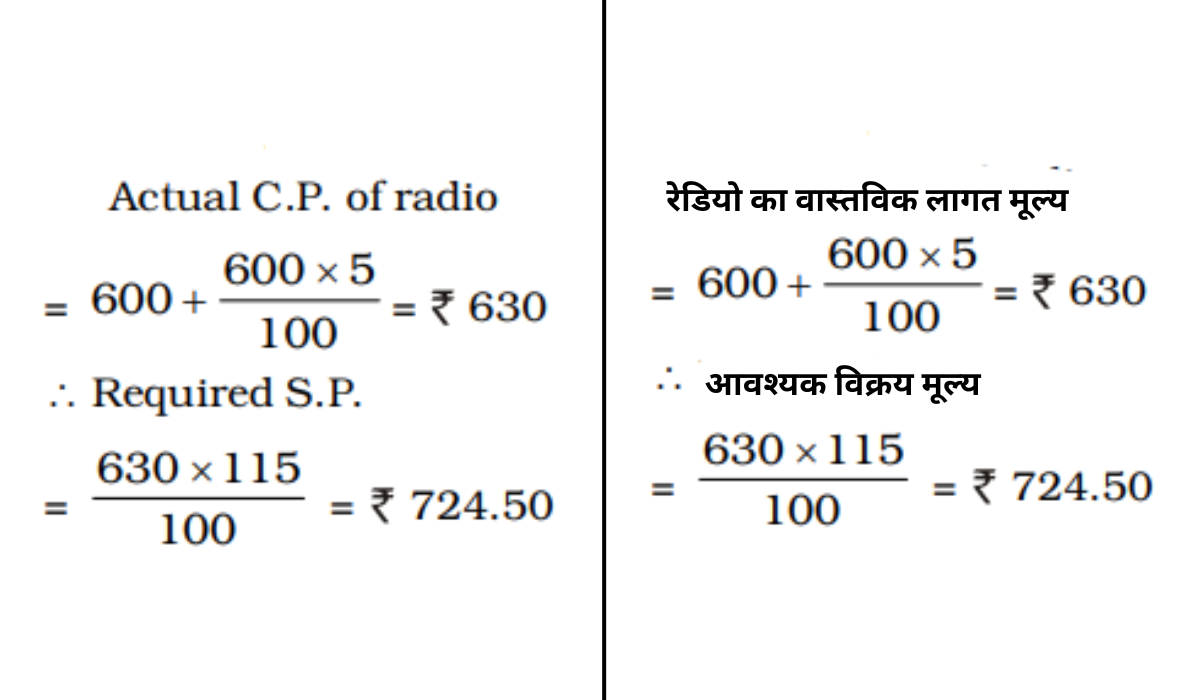Q :

If books bought at prices from ₹150 to ₹ 300 are sold at prices ranging from ₹ 250 to  ₹350, what is the greatest possible profit that might be made in selling 15 books ?

(A) ₹ 750

(B) ₹ 4,250

(C) ₹ 3,000

(D) Cannot be determined

Explanation :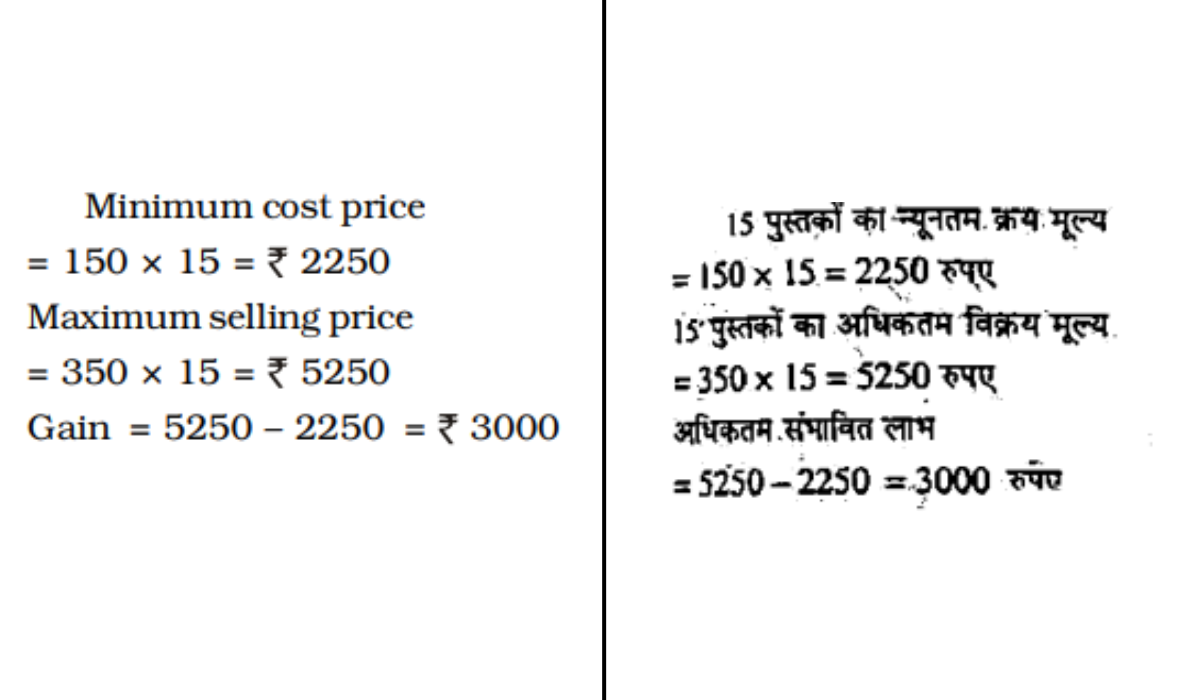Q :

Nisha bought a number of oranges at 2 for a rupee and an equal number at 3 for a rupee. To make a profit of 20% she should sell a dozen for

(A) ₹ 10

(B) ₹ 12

(C) ₹ 6

(D) ₹ 8

Explanation :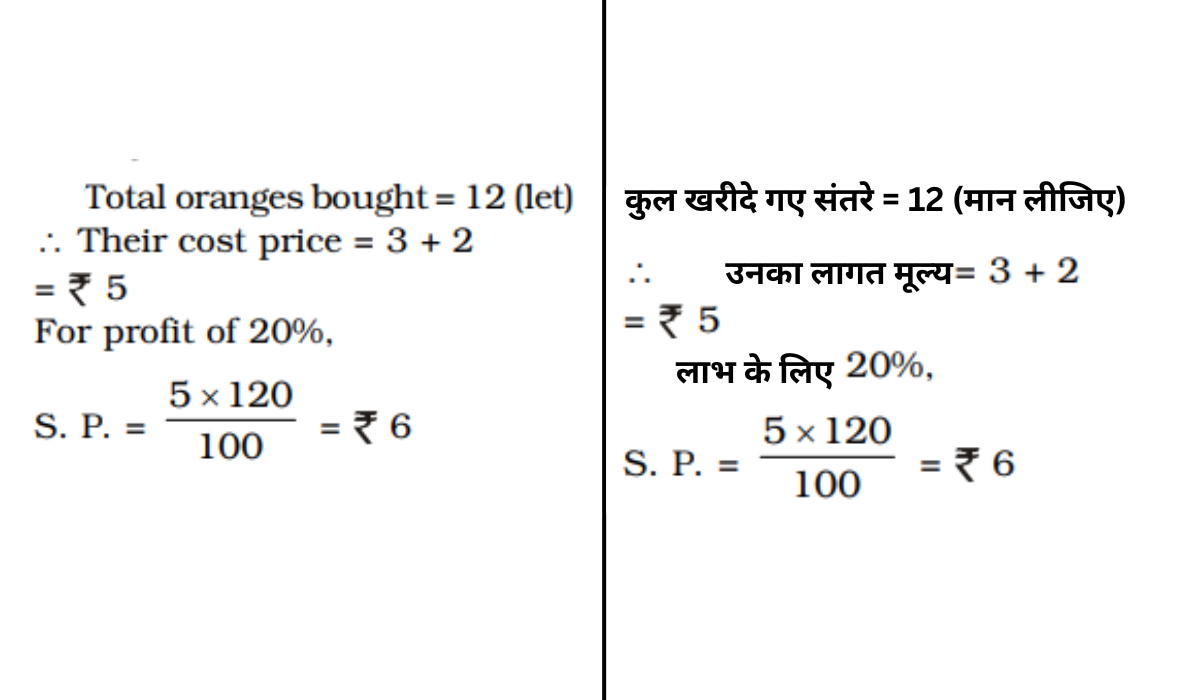Q :

Pooja wants to sell a watch at a profit of 20%. She bought it at 10% less and sold it at ₹ 30 less, but still she gained 20%. The cost price of watch is

(A) ₹ 250

(B) ₹ 225

(C) ₹ 240

(D) ₹ 220

Explanation :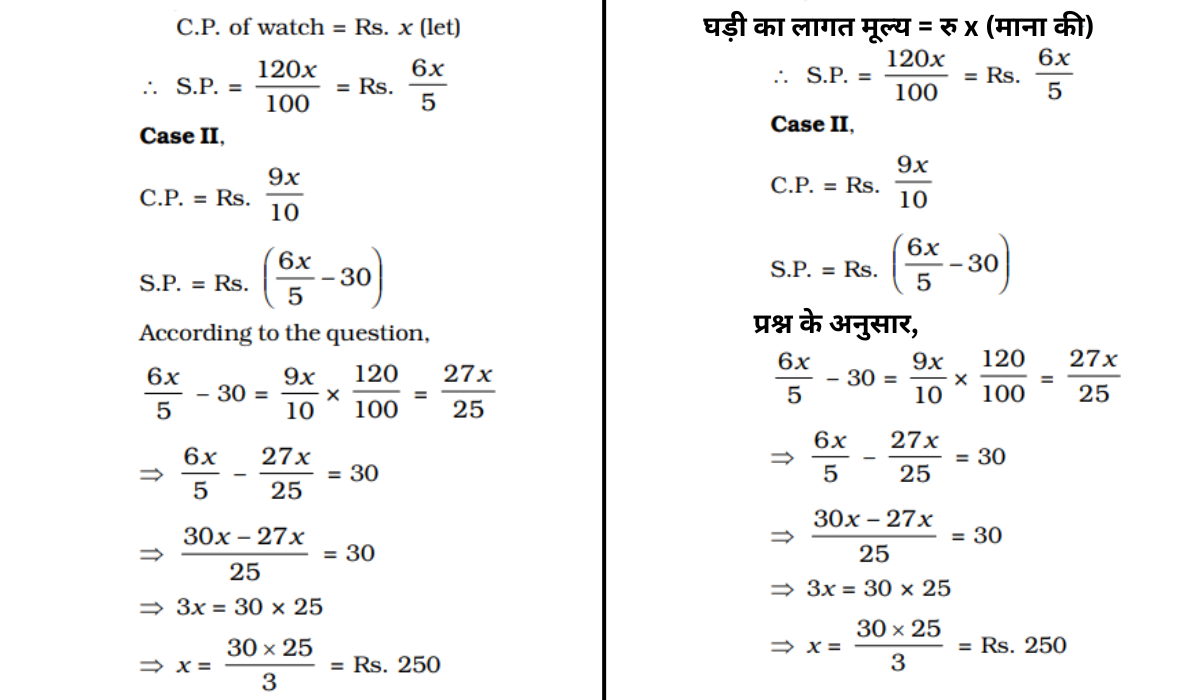Q :

A merchant loses 10% by selling an article. If the cost price of the article is ₹ 15, then the selling price of the article is

(A) ₹ 12.30

(B) ₹ 13.50

(C) ₹ 13.20

(D) ₹ 16.50

Explanation :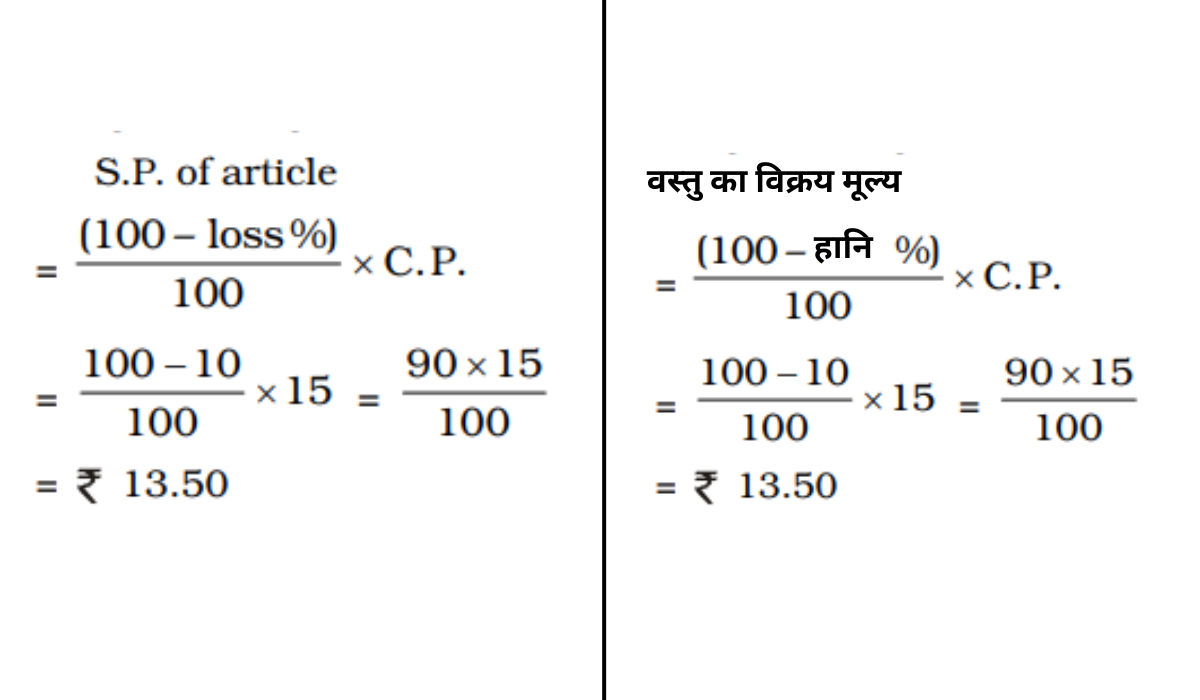Q :

A fruit merchant makes a profit of 25% by selling mangoes at a certain price. If he charges Re. 1 more on each mango, he would gain 50%. At first the price of one mango was

(A) Rs. 4

(B) Rs. 6

(C) Rs. 5

(D) Rs. 7

Explanation :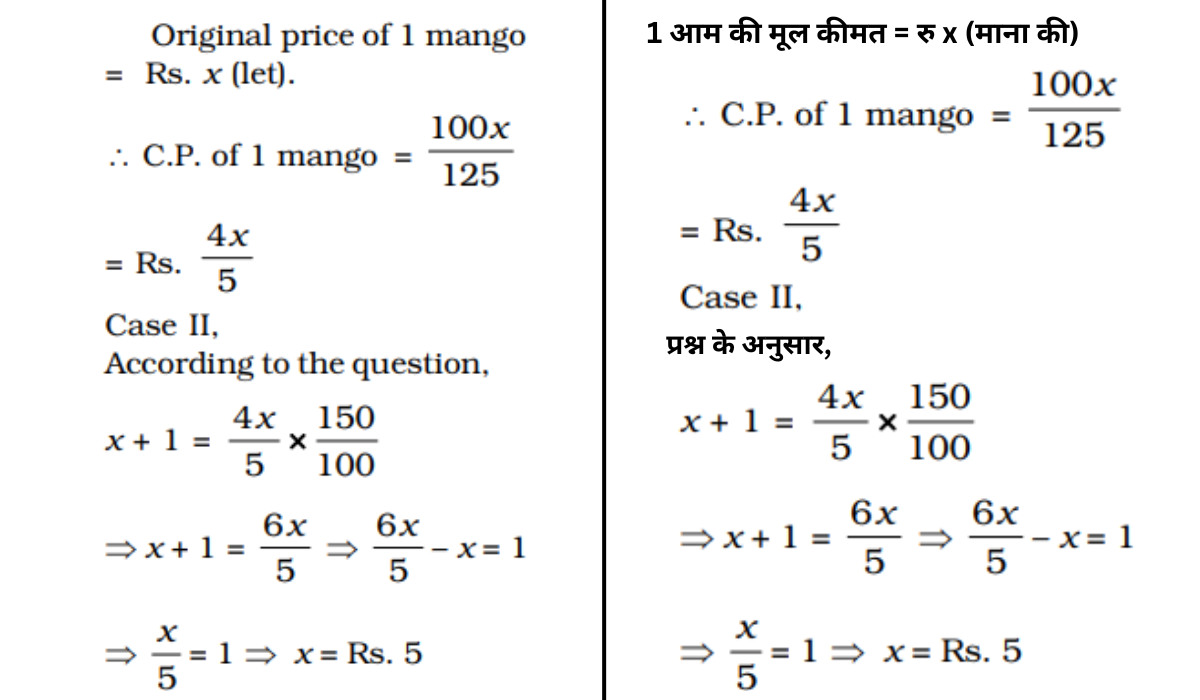Q :

There is 10% loss if an article is sold at Rs. 270. Then the cost price of the article is

(A) Rs 320

(B) Rs. 250

(C) Rs. 300

(D) Rs. 270

Explanation :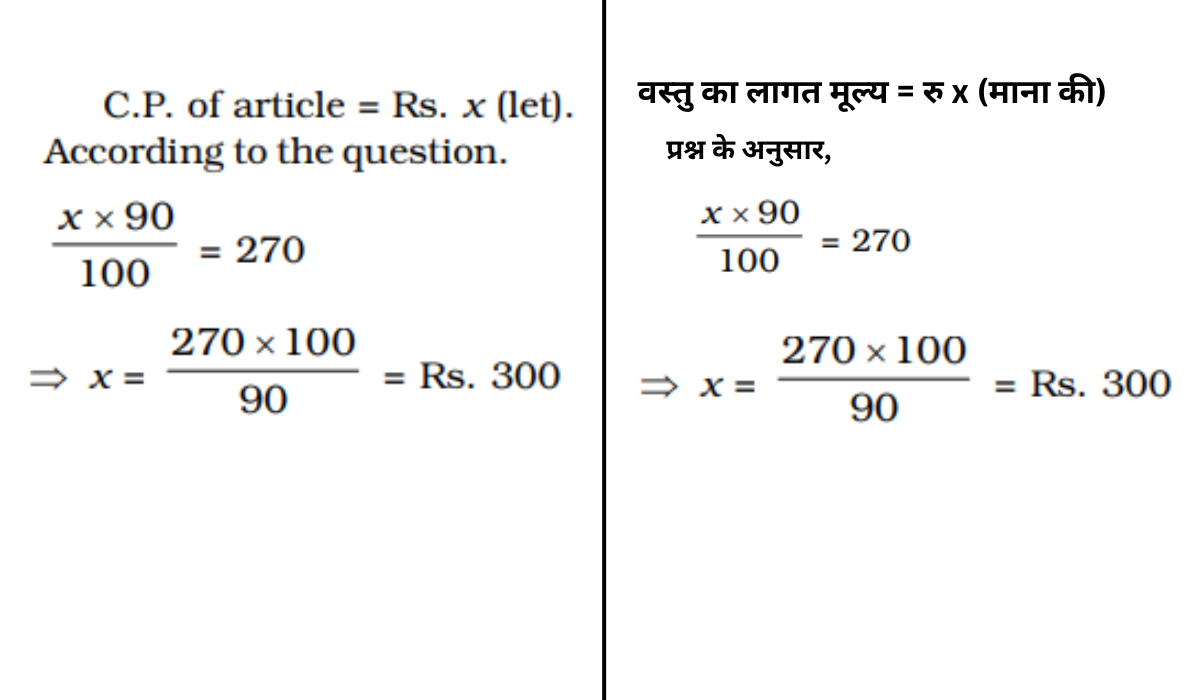Q :

By selling an article for Rs. 450, I lose 20%. For what price should I sell it to gain 20% ?

(A) Rs. 470

(B) Rs. 562.50

(C) Rs. 490

(D) Rs. 675

Explanation :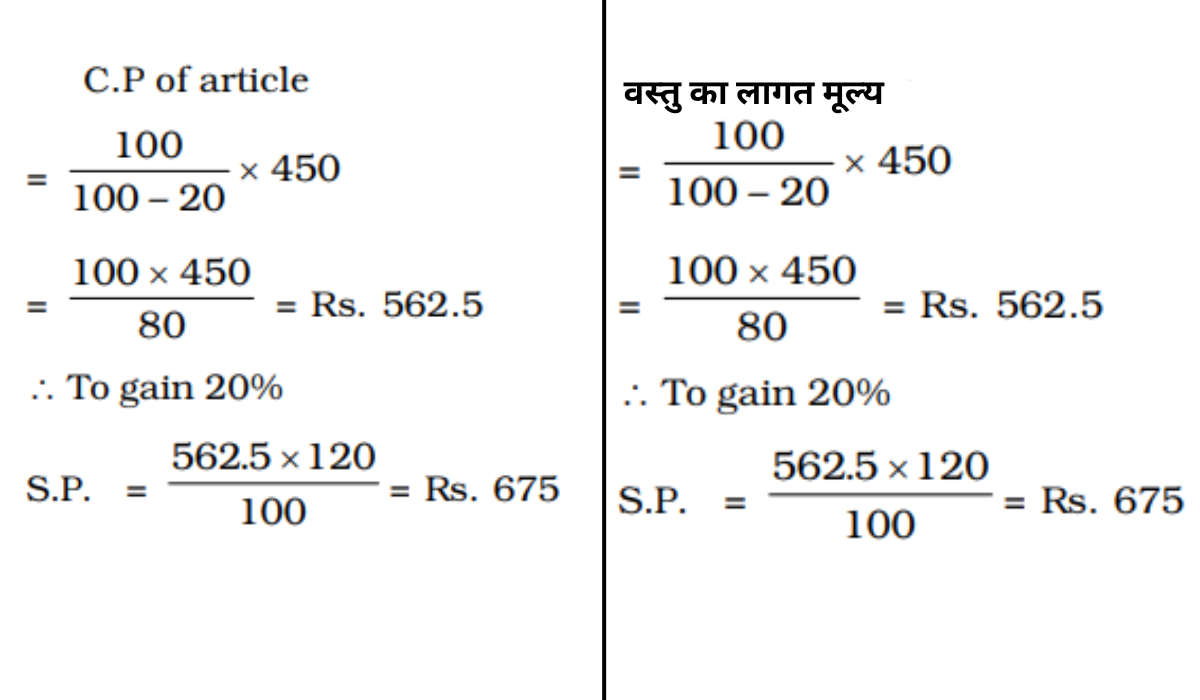Q :

If the profit on selling an article for Rs. 425 is the same as the loss on selling it for Rs. 355, then the cost price of the article is

(A) Rs. 390

(B) Rs. 380

(C) Rs. 400

(D) Rs. 440

Explanation :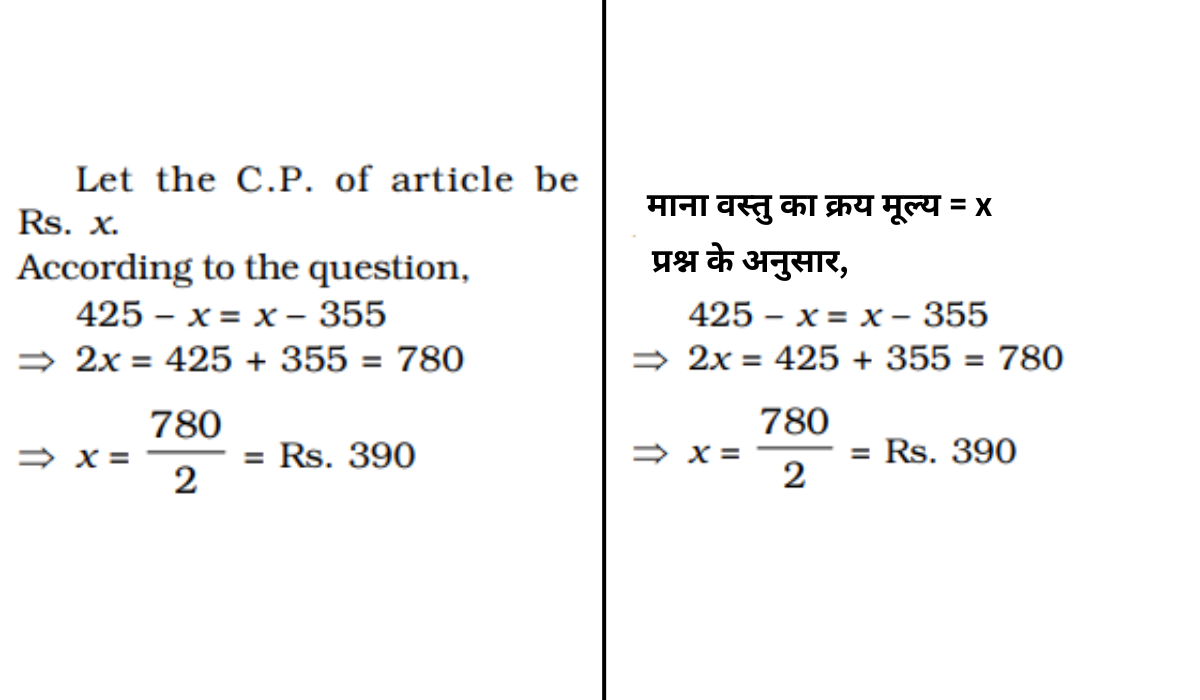Q :

A store sells a watch for a profit of 25% of the cost. Then the percentage of profit against selling price is-

(A) 22%

(B) 20%

(C) 18%

(D) 15%

Explanation :